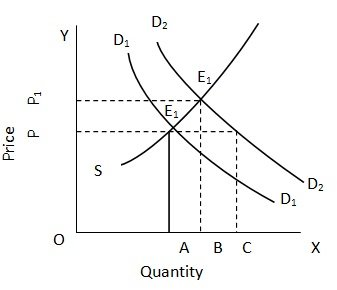Shifts in Demand Curve and Equilibrium Price

# Shifts in Demand Curve & Equilibrium Price Assignment Help

Home / Microeconomics Assignment Help / Shifts in Demand Curve and Equilibrium Price

# Shifts in Demand Curve and Equilibrium Price

Demand for goods can change even without any change in its price. This happens when the income of the consumer or the price complements or substitutes of the goods in question or the tastes and preferences of the consumers undergo a change. If as a result of these changes demand increases, the demand curve will shift upwards and if demand falls it will shift downwards. The impact of such shifts in demand on the equilibrium price can be understood.

The original demand and supply curves respectively. They intersect each other at point E, where demand and supply are equal. EA or OP is the original equilibrium price and OA is the original quantity demanded and supplied in the market. As a result of an increase in demand, the demand curve shifts upwards. D2 D2 is the new demand curve. If the price were to remain at EA or OP, quantity demanded will now be OC but the supply will remain at OA. In other words, demand in this situation will be greater than supply. As a result of this, price will start rising and reach OP1  or E1 B or OP1, will be the new equilibrium price. OB will be both the quantity demanded and supplied in the new situation. Thus, an increase in the demand for a commodity causes an increase in both the equilibrium price and the equilibrium quantity bought and sold.In the event of a decrease in demand, leftward shift in the demand curve, both the equilibrium price and the equilibrium quantity bought and sold will fall. In the diagram above, if the original demand and supply curve are D2 D2 to D1 D1, equilibrium price will fall from E1 B or OP1 to EA or OP and the quantity bought and sold will come down from OB to OA.

### Submit Homework

Submit your homework for a free quote